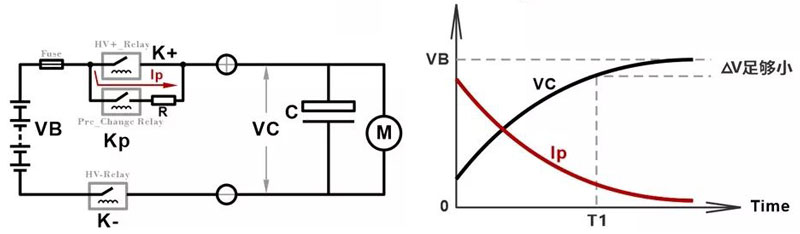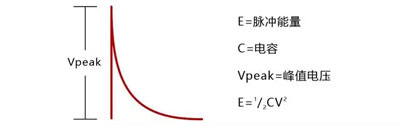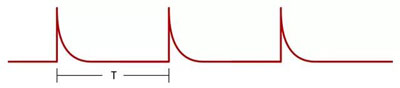T = RC * Ln[(Vbat - V0)/( Vbat - Vpre)]

R=预充电阻

C=负载端电容

Vbat=电池包电压

V0=负载端闭合高压前的电压（可表示为0）

Vpre=预充结束时负载端电压

T = RC * Ln10  则R = T / (C * Ln10)

E = 1/2CV2 = 442J    如果是短时间内连续5个脉冲，总的脉冲能量约为 E总= 442*5